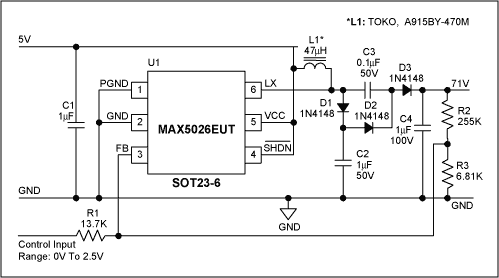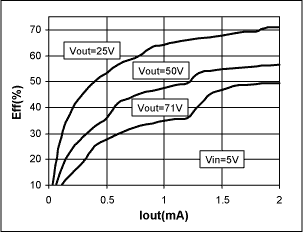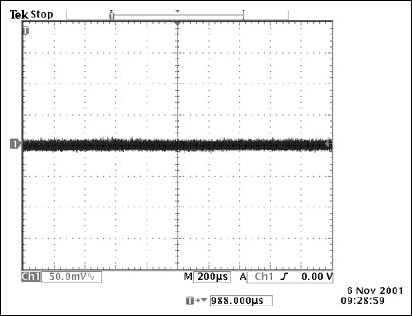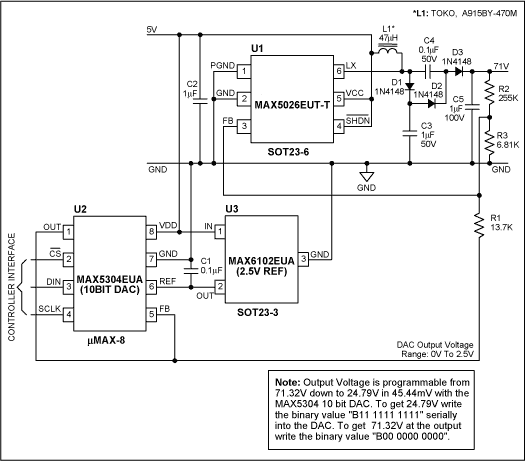# HFAN-03.1.1: Digitally Programmable Low-Noise Avalanche Photo Detector (APD) Bias Circuit

### 要約

A circuit is described to bias an avalanche photodiode as used in fiber-optic receivers. The simple boost converter with voltage-doubler output provides a digitally programmed output voltage of 25V to 71V from a 5V supply. Ceramic filter capacitors and intentionally slowed MOSFET switching transitions provide a low noise output.

The avalanche photodiode detector (APD) is used as a receiver in optical communications, as is the pin diode. The APD is more sensitive, but it must be biased properly to produce the appropriate electron flow for a given flux of photons. Figure 1 shows the schematic of a low-noise APD bias power supply. This circuit is based on a MAX5026 low-noise, fixed frequency PWM boost converter operating in discontinuous inductor current mode.Figure 1. Low-noise APD bias power supply. Varying the control input voltage from 0V to 2.5V results in output voltage change from 71V to 24.7V.

The switching time of this device has been intentionally slowed down to reduce the high-frequency spikes present in other similar devices. The internal switching frequency of the MAX5026 is 500kHz. The internal lateral DMOS switching device has an absolute maximum rating of 40V, and along with the external voltage doubler network formed by C3, D2, D3 and C4, output voltages up to 71V are possible.

Operation of the doubler circuit at steady state is as follows: capacitor C2 transfers charge to C3 during the "ON time" when the LX pin is low (internal DMOS conducting), at the same the inductor L1 is charging up. When the internal DMOS switches off, then current that has built in the inductor forward biases both D1 and D3. Thus, the total voltage presented to capacitor C4 is the sum of VC2 and VC3.

The circuit of Figure 1 is able to provide more than 1mA of output current at 71V output with a 5V input supply. Figure 2 shows the output load regulation at three output voltage settings. Figure 3 shows the output voltage adjustment range with respect to the controlling voltage at the control input. Figure 4 shows the efficiency curves for three output voltage settings.Figure 2. Output voltage regulation vs. output current for three output voltage settings 25V, 50V and 71V.Figure 3. Measured output voltage vs. control input voltage.Figure 4. Efficiency curves.

Figure 5 shows the output ripple at 71V with a 1mA load current at the output with 1µF of ceramic bypass capacitor. The low-noise characteristics of this device are evident from this oscilloscope shot. Output ripple is less than 100mV (see Figure 5).Figure 5. Output voltage ripple. Vout=71V, Iout=1mA. With 1µF output capacitor. Vertical: 50mV/div. Horizontal: 200µs/div.

This can be improved further by placing a low cost Nichicon 10µF, 100V, VX series electrolytic capacitor in parallel with the 1µF ceramic. This results in less than 20mV ripple (see Figure 6).Figure 6. Output voltage ripple. Vout=71V, Iout=1mA. With 10µF electrolytic capacitor added in parallel to the 1µF output capacitor. Vertical: 50mV/div. Horizontal: 200µs/div.

Figure 7 shows how the output of the bias generator can be controlled in steps of approximately 45mV from 25V to 71V by using the MAX5304 10-bit DAC and a 2.5V reference.Figure 7. Low-noise APD bias power supply. Output voltage is digitally programmable from 24V to 71V in steps of 45mV.